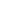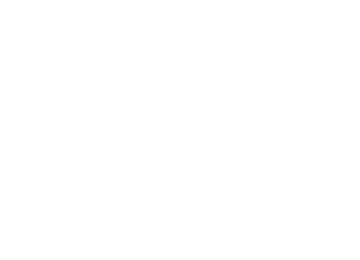### Mock Test 2###### Held on Jun 13, 2021
3:30 AM###### 75 Q’s · 300 marks
180 mins###### Languages
English#### Syllabus

##### Mathematics
• Circles
• Probability
• Limits & Continuity
• Theory of equations
• Mathematical Induction
• Inverse Trigonometric Functions
• Trigonometric Identities & Equations
• Definite Integration
• Binomial Theorem
• Series & Progressions
• Permutations & Combinations
• Straight Lines
• Functions & Graphs

##### Chemistry
• Periodic Table and its Properties
• IUPAC Naming of Organic Compounds
• Ionic Equilibrium
• Acids and Bases
• Coordination Compounds
• Chemical Equilibrium
• General Topics & Mole Concept
• Alkenes & Alkynes Halides
• Alkanes & Alkyl Halides
• Gases & Liquid States
• Redox Reactions
• Chemical Bonding
• Energetics & Thermodynamics

##### Physics
• Motion in a Straight Line
• Solids
• Reflection & Refraction
• Superposition & Reflection
• Uniform Circular Motion
• String Vibration & Air Column
• Laws of Motion & Friction
• Atomic Physics
• Surface Tension & Energy
• Doppler Effect
• Prism
• Viscosity & Terminal Velocity
• Hydrodynamics
• Wave Optics
• Simple Harmonic Motion
• Lenses
• Electric Flux and Gauss's Law